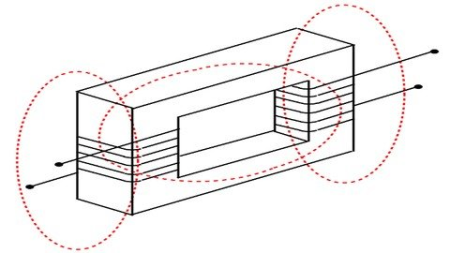Simulation of magnetics for power electronics using PythonMP4 | Video: h264, 1280x720 | Audio: AAC, 44.1 KHzLanguage: English | Size: 5.46 GB | Duration: 16h 36m

Simulation of inductors and transformers using Python Power Electronics
What you'll learn
Basics of magnetic circuits in electrical eeering
Solving basic magnetic circuits
Creating a custom inductor model with magnetic circuit details
Understanding the concept of magnetic coupling
Generating a mathematical model for coupled inductors using basic physics
Simulating coupled inductors
Basics of transformers
Generating a mathematical model for a transformer
Simulating a transformer
Simulating step-up and step-down transformers
Understanding dot polarities of transformer windings
Simulating multi winding transformer windings
Topology and operation of a flyback converter
Simulating a flyback converter
Requirements
Basic Python programming
Basic power electronics knowledge
Simulating power electronic circuits with control loops in Python Power Electronics
Description
This course covers the theory of transformers by simulating them.

The simulation models are built from first principles using fundamental laws of physics. To ease the process of bridging the gap between theory and simulation, we will b with simple inductors and compare simulation results with theory. The course contain several code along sessions with all simulation models built using Python and with the free and open source circuit simulator Python Power Electronics. The final session contains a case study of a flyback converter where besides the theory of operation of the converter, the simulation also covers the high frequency transformer used.
Who this course is for:
Practicing electrical eeers
Power supply eeers